Contents - Index

X-Y-Z Plot

The X-Y-Z Plot command provides 4 options for displaying data of the form Z=f(X,Y)

1)  Isomeric Lines:  displays lines of constant Z in X-Y space (2-D projection)

2)  Color Bands:  uses colors to represent the value of Z in X-Y space (2-D projection)

3 ) Gradient Plot:  uses arrows to show the direction of the gradient with the length of the arrow being proportional to the magnitude of the gradient (2-D projection)

4)  3-D Surface Plot:  displays a 3-D rotatable surface projection using orthogonal or perspective coordinates (available only with the 3-D plotting option selected during setup).

5)  3-D Points Plot: displays an arrays of points (or lines) on a rotatable surface projection using orthogonal or perspective coordinates (available only with the 3-D plotting option selected during setup - Professional version only).

Note that all five types of X-Y-Z plots have additional options that can be viewed or changed after the plot is constructed by right-clicking on the plot to bring up the Modify Plot dialog.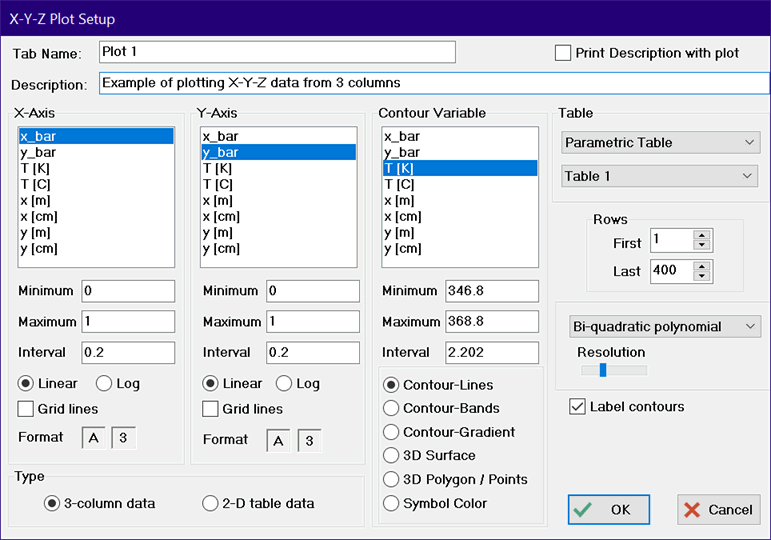A contour plot requires three-dimensional data for construction.  The data may be provided either in 3 separate columns for the X, Y, and Z variables or in a 2-D table.  In either case, the data used to construct the plot can be from the Parametric, LookupArrays or Integral table windows.  The table from which the data are taken is selected from the drop-down controls at the upper right of the dialog.

If the 3-column data radio button is selected, EES will expect that three dimensional data to be provided in three columns from one of the table windows.  The X, Y, and Z variables are then selected from the three lists.  Data provided in this form need not be in any particular order.   If the 2-D table data radio button is selected, EES will assume the column numbers in the table are proportional to the X-coordinate whereas the rows are proportional to the Y-coordinate.  If the Transpose button is checked, the the column numbers in the table will be propostional to the Y-coordinate and the rows will be proportional to the X-coordinate. The Z-values will be read from the table.  Controls are provided to allow a subset of rows and/or columns in the table to be plotted.  The X and Y axis scaling is, by default, set to 0 to 1, but it can be changed as desired for the axis scale display.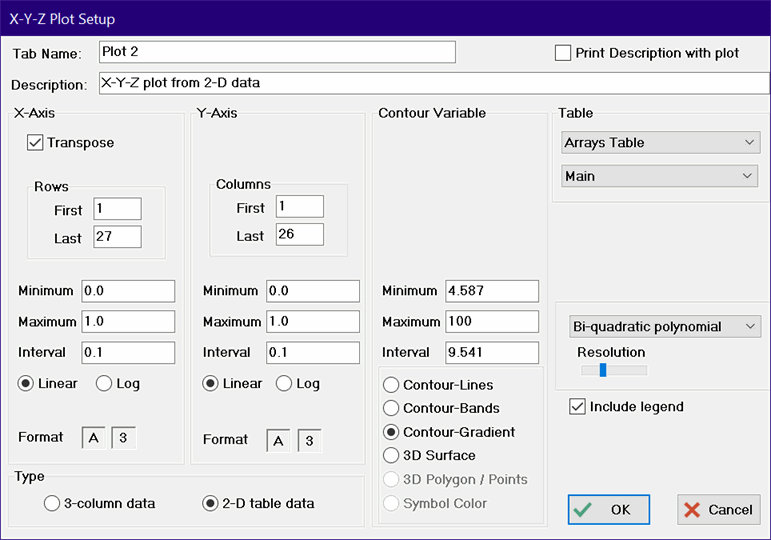To prepare the X-Y-Z plot for all of the options except the 3-D points plot, the selected data must be interpolated or extrapolated as necessary to provide values of variable Z over the entire range of X and Y at relatively fine intervals.  Two different algorithms are provided to do this gridding.  The choice of gridding algorithm is made from the drop down list at the lower right of the dialog box.  The grid size is controlled by the Resolution slider control.  When the slider is positioned at its left-most point, the grid size is 50 by 50 units.  At the right-most point, the grid size is 100 by 100.

With this approach, the selected data are first scaled and then fitted to a function of the form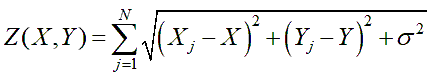where N is the number of data points, the w values are weighting factors, and s is a relative smoothing parameter which, based on experimentation, is best set to zero for numerically-generated data.  However smoothing may be needed for experimental data or for sparse data sets.  Moving the Smoothing slider bar to the right increases s which increases the smoothing effect.

The weights are found by solving the linear system of N equations that force the above equation to perfectly represent the provided data.  Once the weights are determined the equation is used to interpolate or extrapolate the values of Z to particular values of x and y.  The computations needed to determine the weights are O(N cubed) so there is a practical upper limit to the number of points (N) that can be accommodated.  This upper limit is controlled by the Resolution slider.  If more data points are provided than are specified by the Resolution slider, EES will select a subset of the data using a clustering algorithm.  At full resolution, you may have to wait a while for the plot to be prepared depending on the speed of your computer.

With this approach the data are fitted to a second-order polynomial of the form: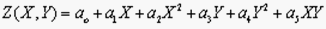A separate set of the a coefficients is fitted for each point in the grid using weighted linear least squares.  The number of grid points, controlled by the setting of the Resolution slider, ranges from 2,500 to 10,000 .

Two-dimensional contour plots may be produced using isometric lines or color bands representing constant values of Z.  The number of lines or bands is determined by as the difference between the maximum and minimum values divided by the interval value.  Each isometric line is a separate plot overlay, whereas the color band plot is simply one plot.  A maximum of 25 isometric lines and 250 colors is allowed.  Note that selecting a large number of colors (>100) results in a plot that has nearly continuous colors.  The contours can be displayed in shades of gray, or colors range from red to blue or using the full spectrum.  The choice of color scheme can be changed in the Modify Contour/Gradient Plot dialog.   ranging from blue (low values) to red (high values).  The resulting plot can be quite pretty, but the time required to display the plot will increase with the number of colors.  A Label Contours check box control is provided for isometric contour plots.  In this case, EES will generate a text item containing the value for each contour line and place it on the line.  These text items can be moved or changed just as any other Plot window text items.   If a banded color plot is chosen, an Include Legend check box is provided.  If the Include Legend check box is selected, a color legend will be displayed to the right of the plot relating colors to the values of Z.  Note that, on a color band contour plot, you can read the value of Z directly in the Plot Window title bar at any X-Y position by holding the Ctrl and Shift keys down and moving the mouse.  An X-Y or bar plot can be overlaid on 2 two-dimensional contour plot using the Overlay Plot command.

The three dimensional plotting package is based on a modified version of the public domain GLScene package (http://www.glscene.org/). The plot has been modified to work within EES and to optionally display values of a 4th dimension with color or gray scale for the surface 3-D plot.  For example, it is possible to display the temperature as a color on an X-Y-Z surface.  The color variable is selected by clicking on header for the Z-Axis variable.  This action will cause the list to display the Color variable.  The color variable list is shown in yellow to make the distinction clear.  If a color variable is not selected, it is assumed to be the same as the Z-axis variable.  After it is constructed, the three-dimensional plot can be rotated about its center point by pressing the mouse button on the plot and dragging it in the desired direction.  A number of controls are provided to, for example, scale the image, set colors, and display grids and surface grid lines.  These controls are provided on the 3-D plot control panel.  Only 1 3-D surface plot can appear but the Professional version allows 3-D points to be overlayed on the 3-D surface plot, provided that the 3-D surface plot is the first plot in the plot window.

The 3-D points plot will plot a three-dimensional representation of data provided in three separate columns of any of the tables.  This options is only available in the Professional version.  Clicking the 3-D Points button will change the display as shown in the following figure.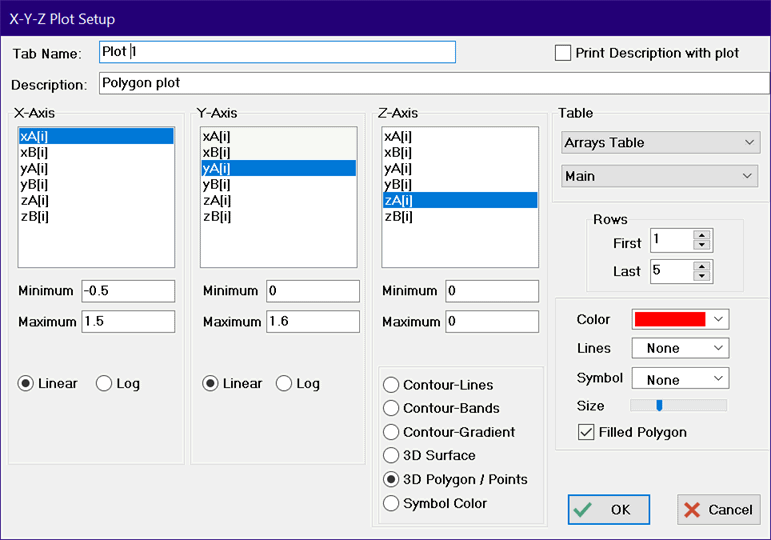The minimum and maximum values for the X, Y and Z axes can be specified.  Only points within the specified ranges will be plotted.  The color, line type, symbol and symbol size can be specified.  Overlays are permitted for the 3-D points plot.  In addition, the 3-D points plot can be overlayed on a 3-D surface plot.  Once the plot has been constructed, there are a number of additional controls on the 3-D plot control panel that can be changed to affect the appearance of the plot.